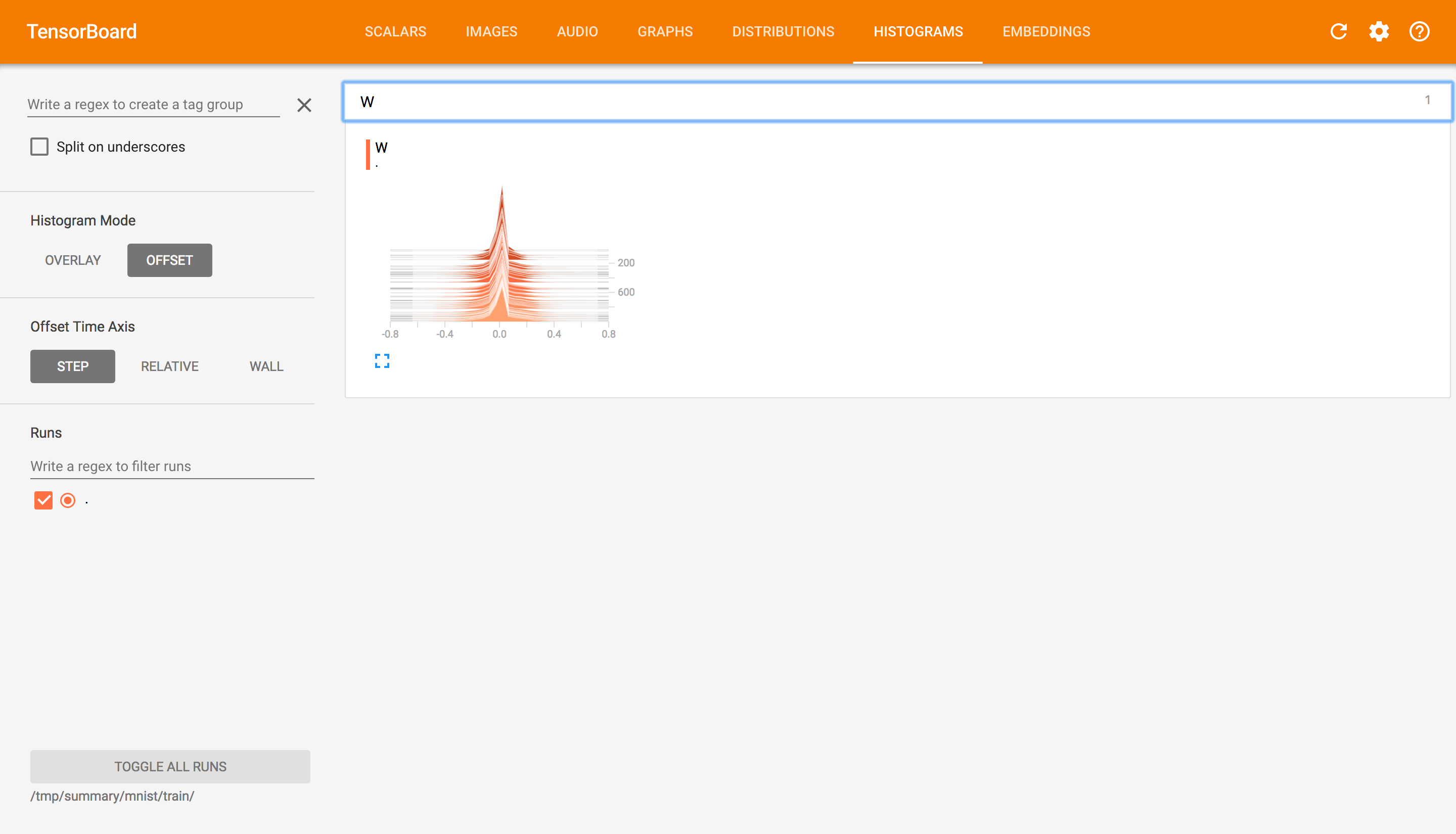# 可视化学习Tensorboard

TensorBoard 涉及到的运算，通常是在训练庞大的深度神经网络中出现的复杂而又难以理解的运算。为了更方便 TensorFlow 程序的理解、调试与优化，发布了一套叫做 TensorBoard 的可视化工具。你可以用 TensorBoard 来展现你的 TensorFlow 图像，绘制图像生成的定量指标图以及附加数据。## 数据序列化-events文件

TensorBoard 通过读取 TensorFlow 的事件文件来运行。TensorFlow 的事件文件包括了你会在 TensorFlow 运行中涉及到的主要数据。事件文件的生成通过在程序中指定tf.summary.FileWriter存储的目录,以及要运行的图

tf.summary.FileWriter('/tmp/summary/test/', graph=default_graph)


## 启动TensorBoard

tensorboard --logdir=path/to/log-directory


## 节点符号import tensorflow as tf

graph = tf.Graph()

with graph.as_default():
with tf.name_scope("name1") as scope:
a = tf.Variable([1.0,2.0],name="a")
with tf.name_scope("name2") as scope:
b = tf.Variable(tf.zeros(),name="b")
c = tf.Variable(tf.ones(),name="c")
with tf.name_scope("cal") as scope:
d = tf.concat([b,c],0)

with tf.Session(graph=graph) as sess:
tf.global_variables_initializer().run()
# merged = tf.summary.merge_all()
summary_writer = tf.summary.FileWriter('/tmp/summary/test/', graph=sess.graph)
sess.run([d,e])## 添加节点汇总操作

• tf.summary.scalar() 收集对于损失函数和准确率等单值变量
• tf.summary.histogram() 收集高维度的变量参数
• tf.summary.image() 收集输入的图片张量能显示图片

cross_entropy = tf.reduce_mean(tf.nn.softmax_cross_entropy_with_logits(labels=y_label, logits=y))
correct_prediction = tf.equal(tf.argmax(y, 1), tf.argmax(y_label, 1))
accuracy = tf.reduce_mean(tf.cast(correct_prediction, tf.float32))

tf.summary.scalar("loss",cross_entropy)

tf.summary.scalar("accuracy", accuracy)

tf.summary.histogram("W",W)


# 合并
merged = tf.summary.merge_all()
summary_writer = tf.summary.FileWriter(FLAGS.summary_dir, graph=sess.graph)

# 运行
summary = sess.run(merged)

#写入